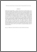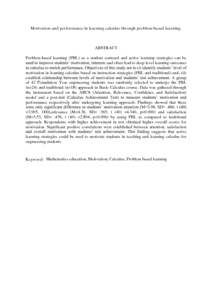# Motivation and performance in learning calculus through problem-based learning.

## Citation

Mokhtar, Mohd Zin and Ahmad Tarmizi, Rohani and Mohd. Ayub, Ahmad Fauzi and Nawawi, Mokhtar (2013) Motivation and performance in learning calculus through problem-based learning. International Journal of Asian Social Science, 3 (9). pp. 1999-2005. ISSN 2226-5139; ESSN: 2224-4441

## Abstract

Problem-based learning (PBL) as a student centered and active learning strategies can be used to improve students’ motivation, interests and often lead to deep level learning outcomes in calculus to enrich performance. Objectives of this study are to (i) identify students’ level of motivation in learning calculus based on instruction strategies (PBL and traditional) and; (ii) establish relationship between levels of motivation and students’ test achievement. A group of 42 Foundation Year engineering students was randomly selected to undergo the PBL (n=24) and traditional (n=18) approach in Basic Calculus course. Data was gathered through the instrument based on the ARCS (Attention, Relevance, Confidence and Satisfaction) model and a post-test (Calculus Achievement Test) to measure students’ motivation and performance respectively after undergoing learning approach. Findings showed that there were only significant difference in students’ motivation attention (M=3.98, SD= .486; t (40) =3.905, .000),relevance (M=4.36, SD= .365; t (40) =4.340, p=0.000) and satisfaction (M=3.53, SD= .436; t (40) =2.894, p=0.006) by using PBL as compared to traditional approach. Respondents with higher achievement in test obtained higher overall scores for motivation. Significant positive correlations were established between attention, satisfaction and overall motivation with students’ test achievement. This finding suggests that active learning strategies could be used to motivate students in teaching and learning calculus for engineering students.Preview
PDF (Abstract)
Motivation and performance in learning calculus through problem.pdfView Item